# LOW CURRENT PROCESS OF WATER ELECTROLYSIS

Ph. M. Kanarev

E-mail: kanphil@mail.kuban.ru

Low voltage process of water electrolysis is known from Faraday’s times. It is widely used in modern industry. Voltage of 1.6-2.3 volts is operation voltage between the anode and the cathode of the electrolyzer; current strength is tens and hundreds of amperes. In accordance with Faraday’s law, energy consumption for production of one cubic meter of hydrogen is nearly 4 kWh  in this case.

The analysis of the water molecule structure (Fig. 1) worked out by us shows the possibility of water electrolysis at minimal current and even without it. The protons of the hydrogen atoms in water molecules can be combined with each other and can form clusters. As a result, an orthohydrogen molecule is formed (Fig. 2). A question arises: is it possible to separate this molecule from such cluster? A search of the answer to this question has lasted nearly three years. Its results are given in Tables 1, 2 and 3.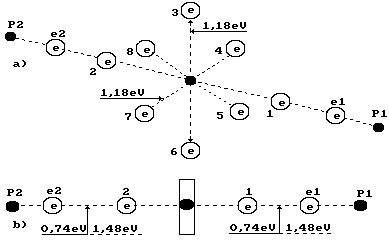Fig. 1. Water molecule diagram:

1,2,3,4,5,6,7,8 are numbers of the electrons of the oxygen atom; P1, P2 are the hydrogen atom nuclei (the protons); e1 and e2 are the electron numbers of the hydrogen atoms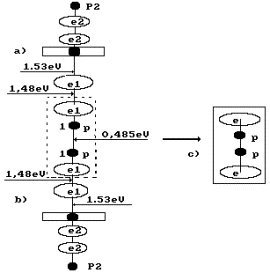Fig. 2. Formation diagram of  orthohydrogen

a) and b) water molecule diagrams; c) orthohydrogen

It is known that a gram-atom is equal to atomic mass of substance; a grammolecule is equal to molecular mass of substance. For example, the grammolecule of hydrogen in the water molecule is equal to two grams; the gram-atom of the oxygen atom is 16 grams. The grammolecule of water is equal to 18 grams. Hydrogen mass in a water molecule is 2x100/18=11.11%; oxygen mass is 16x100/18=88.89%; this ratio of hydrogen and oxygen is in one liter of water. It means that 111.11 grams of hydrogen and 888.89 grams of oxygen are in 1000 grams of water.

One liter of hydrogen weighs 0.09 g; one liter of oxygen weighs 1.47 g. It means that it is possible to produce 111.11/0.09=1234.44 liters of hydrogen and 888.89/1.47=604.69 liters of oxygen from one liter of water. It appears from this that one gram of water contains 1.23 liters of hydrogen. Energy consumption for production of 1000 liters of hydrogen is 4 kWh and for one liter 4 Wh. As it is possible to produce 1.234 liters of hydrogen from one gram of water, 1.234x4=4.94 Wh is spent for hydrogen production from one gram of water now.

Instruments and equipment used during the experiment

Special experimental low current electrolyzer (Fig. 3); voltmeter of the highest accuracy class (accuracy class of 0.2 GOST 9711-78); ammeter of the highest accuracy class (accuracy class of 0.2 GOST 9711-78)’ electronic scale with scale division value of 0.1 and 0.01 g; stop watch with scale division value of 0.1 s.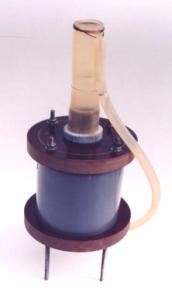Fig. 3. Low current electrolyzer in the closed form (in the process of patenting)

The author expresses acknowledgement to A.I. Tlishev, candidate of technical sciences, for production of this experimental device and other ones and their testing.

Experimental results

Table 1

 Indices Sum 1 - duration of the experiment  t, h 6.000 2 – readings of voltmeter V, volts 3.750 3 – ammeter readings I, amperes 0.020 4 – power P, watts hour (P=VxIxτ/60) 0.450 5 – continue of experiment without input energy in 6 series, min 0.000 6 – mass difference, grams 0.52 7 – mass of evaporated water, grams 0.01x6=0.06 8 – mass of water converted in hydrogen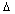m, grams 0.46 9 – specific power P’=P/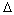m, Watt/gram of water 0.98 10 – existing  specific power P’’, Watt/gram of water 4.94 11 – the reducing  power on the production of hydrogen, times K=P’’/P’ 5.04 12– quantity of released hydrogen, ΔМ =0.46x1.23x0.09=0.051, grams 0.051 13 – energy content of hydrogen being obtained      (Е=0.051х142/3,6)=2.008 Wth 2.008 14- energy efficacy of low ampere process of water electrolysis (Eх100/P), % 446.2

Note: In Table 1, the results of the experiment are given when frequency of nearly 500 Hz has been generated in the power supply

Table 2

 Indices Sum 1 - duration of the experiment with input energy in 6 series t, min 6x30=180.0 2 – readings of voltmeter V, volts 3.750 3 – ammeter readings I, amperes 0.022 4 – power P, watts hour (P=VxIxτ/60) 0.247 5 – continue of experiment without input energy in 6 series, min 6x30=180.0 6 – mass difference, grams 0.45 7 – mass of evaporated water, grams 0.1x6=0.06 8 – mass of water converted in hydrogen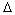m, grams 0.39 9 – specific power P’=P/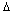m, Watt/gram of water 0.63 10 – existing  specific power P’’, Watt/gram of water 4.94 11 – the reducing  power on the production of hydrogen, times K=P’’/P’ 8.40 12– quantity of released hydrogen, ΔМ =0.39x1.23x0.09=0.043, grams 0.043 13 – energy content of hydrogen being obtained      (Е=0.043х142/3,6)=1.70 Wth 1.70 14- energy efficacy of low ampere process of water electrolysis (Eх100/P), % 689.0

Note: In Table 2, the results of the experiment are given when no additional frequency has been generated by the power supply

Table 3

 Indices Sum 1 - duration of the experiment with input energy in 6 series t, min 6x5=30 2 – readings of voltmeter V, volts 13.60 3 – ammeter readings I, amperes 0.020 4 – power P, watts hour (P=VxIxτ/60) 0.136 5 – continue of experiment without input energy in 6 series, min 6x55=330 6 – mass difference, grams 0.44 7 – mass of evaporated water, grams 0.01x6=0.06 8 – mass of water converted in hydrogenm, grams 0.38 9 – specific power P’=P/m, Watt/gram of water 0.358 10 – existing  specific power P’’, Watt/gram of water 4.94 11 – the reducing  power on the production of hydrogen, times K=P’’/P’ 13.80 12– quantity of released hydrogen, ΔМ =0.38x1.23x0.09=0.042, grams 0.042 13 – energy content of hydrogen being obtained      (Е=0.042х142/3,6)=1.66 Wth 1.66 14- energy efficacy of low ampere process of water electrolysis (Eх100/P), % 1220.0

Note: In Table 3, the results of the experiment are given when frequency of nearly 500 Hz has been generated in the power supply

First of all, we should note that the anode and the cathode are made of one and the same material: steel. It excludes the possibility of formation of a galvanic cell. If we analyze Tables 1, 2 and 3, we’ll see the electrolysis process takes place at very low current of 0.02 A; that’s why it has been called low current one. Further, this process consisted of two cycles in some experiments; in one cycle, the electrolyzer is connected to the power line; in another cycle, it is disconnected.

Gas generation process is manifested by release of the bubbles being formed. The bubbles go on being released after the electrolyzer is disconnected from the supply line (Tables 2 and 3). When the electrolyzer is de-energized, gas release intensity is reduced, but it is not stopped during many hours. It is proved by the fact that electrolysis takes place at the expense of potential difference on the electrodes. It should be noted that small potential difference takes place on the electrodes of the empty electrolyzer and immediately after it has been charged with electrolyte prior to its connection to the supply line.

If water electrolysis took place only at the expense of the electrons emitted by the cathode, i.e. according to Faraday’s law, current value would be greater and the stock of these electrons would give out after the electrolyzer was de-energized and the gas release process would be stopped.

After electrolyzer de-energizing, gas release during a long period of time proves the fact that the molecules of oxygen and hydrogen are formed without the electrons emitted by the cathode, i.e. at the expense of the electrons of the water molecule itself. Let us analyze this process  in detail.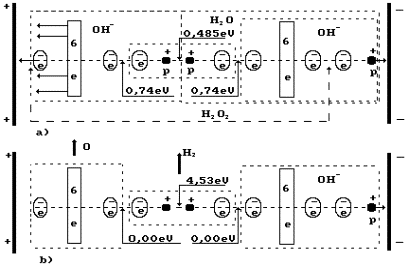Fig. 4. Diagram of low current electrolysis process

In Fig. 4, the anode is shown leftward and the cathode is shown rightward. The proton of the hydrogen atom in water molecule is oriented to the cathode; another proton of this molecule is connected with the proton of the ion OH`  (leftward). As a result,  a cluster chain is formed; rightward, the water molecule H2O is situated; leftward, the ion OH`    is situated (Fig. 4, a); the orthohydrogen molecule H2 is in the center (Fig. 4, a, b). Both protons of the hydrogen molecule are connected by energy 0.485 eV corresponding to water molecule cluster formation energy at the temperature of 20°C. Binding energies of the left electron of the hydrogen molecule with the electron of the oxygen atom and the right electron with the electron of the ion OH`    are equal to 0.74 eV (Fig. 1).

Thus, the complex cluster chains with strict orientation between the anode and the cathode are formed in the electrolytic solution under the influence of the electrostatic field.

Let us pay attention to the fact that the axis electron of the oxygen atom  and its six ring electrons of the ion OH`    are attracted to the anode simultaneously (Fig. 4, a). Electrostatic forces attracting six ring electrons to the anode deform the electrostatic field in such a way that the axis electron comes to the nucleus of the oxygen atom, and six ring electrons withdraw from the atomic nucleus. As the electron withdrawal process from the atomic nucleus is an endothermic one, six ring electrons absorb 1.18 eV x 6 = 7.08 eV (s. Fig. 5). It will automatically transform both axis electrons of the oxygen atom to the energy levels corresponding the excited state of the oxygen atom. Energy absorbed by the ring electrons of two atoms of oxygen is 7.08 x 2 = 14.16 eV.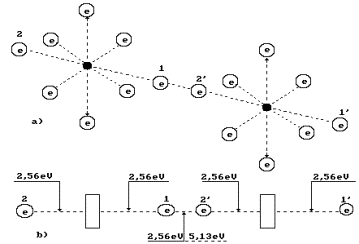Fig. 5. Diagram of binding energy distribution between the electrons in the oxygen molecule

When two oxygen atoms have been separated from two cluster chains, their two axis electrons form a covalent bond releasing 5.13 eV of energy (s. Fig. 5). Other two electrons of the oxygen atoms arranged at the ends of the axis of the molecule O2 will go to the energy levels with binding energies of 2.56 eV having emitted (2.56-0.74) x 2 = 6.92 eV.

Hydrogen molecule fusion energy is (4.53-0.485-1.48x2)=1.085 eV taking into consideration a binding energy change between its protons and electrons (Fig. 4) and conversion of orthohydrogen into parahydrogen. Fusion energy of two molecules of parahydrogen is 1.085 x 2 = 2.170 eV. As a result, total energy of fusion of one oxygen molecule and two hydrogen molecule is (5.13+6.92+2.170)=14.22 eV.

Difference between absorbed energy and emitted energy will be (14.22-14.16)=0.06 eV. This is exothermic energy. If we take into account that nearly 0.5 liter of hydrogen has been released during the experiment, thermal energy should be generated.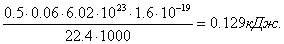(1)

Solution mass in the electrolyzer was nearly 0.3 kg. If we take it into consideration, the solution should be heated by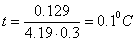(2)

The experiment has shown that when the electrolyzer is energized, solution temperature is increased by 1.5-2°C. When the electrolyzer is de-energized and the electrolysis process goes on, solution temperature is reduced. When the electrolyzer is de-energized, potential on the electrodes is reduced gradually. It means that the initial stock of the electrons on the cathode is reduced as well. It takes place due to restoration of the ions of an alkaline metal. When the ions are restored, they take electrons from the cathode and reduce potential between the electrodes. It is manifested by cathode surface color. It acquires color of the alkaline metal being used.

Thus, low ampere electrolyzer can operate in two modes: connected to the supply line and disconnected from it. When the electrolyzer is connected to the supply line, a part of gases is released using the electrons of the cathode, and a part is released without the use of these electrons. When the electrolyzer is disconnected from the supply line, the cathode electrons are spent for restoration of alkaline metals.

In Fig. 4, a, the boundaries of the hydrogen peroxide molecule H2O2 are shown in the cluster chain (Fig. 6). It is clear (Fig, 4) that the hydrogen peroxide molecule is released only when a hydrogen atom being in contact with the cathode is released from the cluster chain. It takes place when voltage is increased. Hydrogen peroxide is released and interacts with the anode material; if it is iron, foam with red flakes is formed at once. Foam is an apparent feature of spontaneous gas release process disturbance.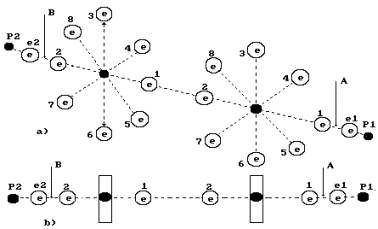Fig. 6. Diagram of the model of hydrogen peroxide H2O2

Involuntarily, the results being obtained form an aspiration to find an analogy of the described low ampere process of water electrolysis in Nature.

It is known that carbon dioxide CO2 is absorbed during photosynthesis. It is considered that carbon C of the molecule CO2  is used for plant cell construction, and oxygen O2 is released. Now we have every reason to doubt at it and to suppose that the molecule CO2  is used totally for plant cell construction. Water molecules release oxygen; the hydrogen atoms of water molecules are used as connecting links of the molecules, from which the plant cells are constructed. This process is similar to the process shown in Fig. 4.

CONCLUSION

Simplicity and 100% reproducibility of the experiments being described afford ground for the fact that mankind has got a chance to avoid energy famine and environmental crisis.

REFERENCES

1. Ph.M. Kanarev. The Foundation of Physchemistry of Microworld. Krasnodar, 2002. 320 pages.

2. Ph.M. Kanarev. The Foundation of Physchemistry of Microworld. The second edition (in Russian) hppt://www.ikar.udm.ru/sb28-2.htm

3. Ph.M. Kanarev. The Foundation of Physchemistry of Microworld. The second edition (in English). http://book.physchemistry.innoplaza.net

Webmaster: j_hartikka@hotmail.com

Low Current Process of Water Electrolysis by Prof. Kanarev:

http://Kanarev.lowcurrent.innoplaza.net

http://hydrogen.innoplaza.net

<< Kanarev´s Page